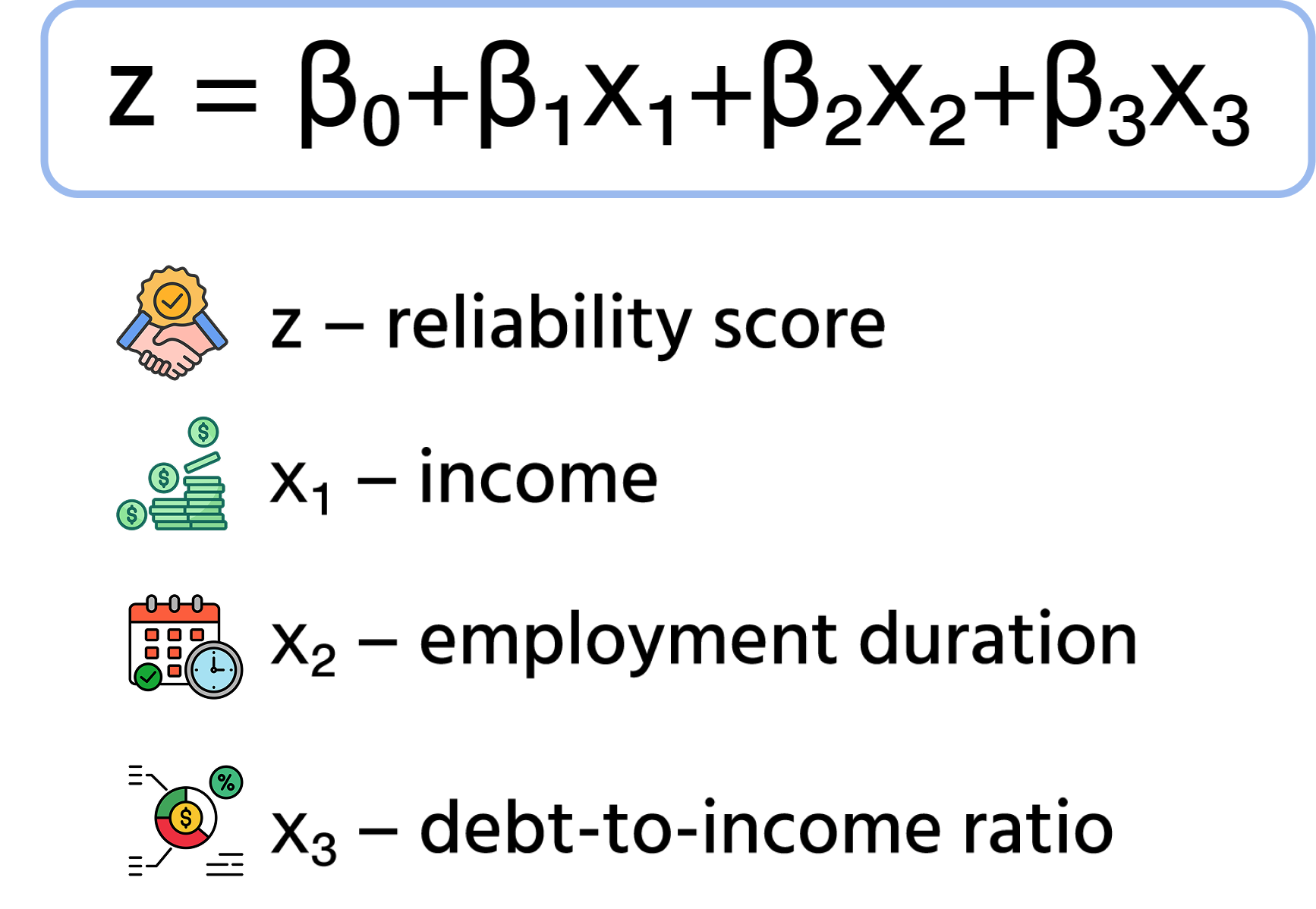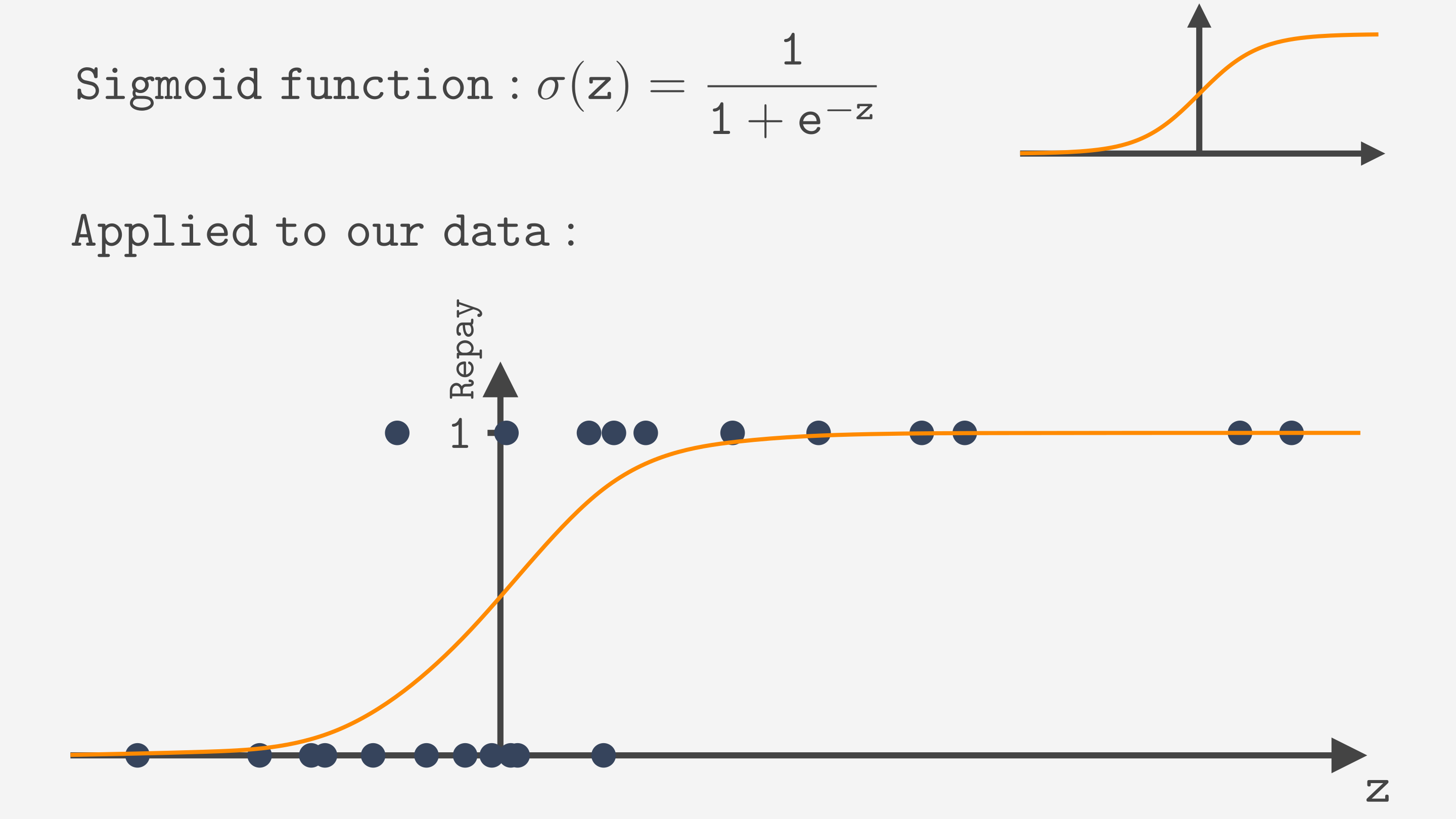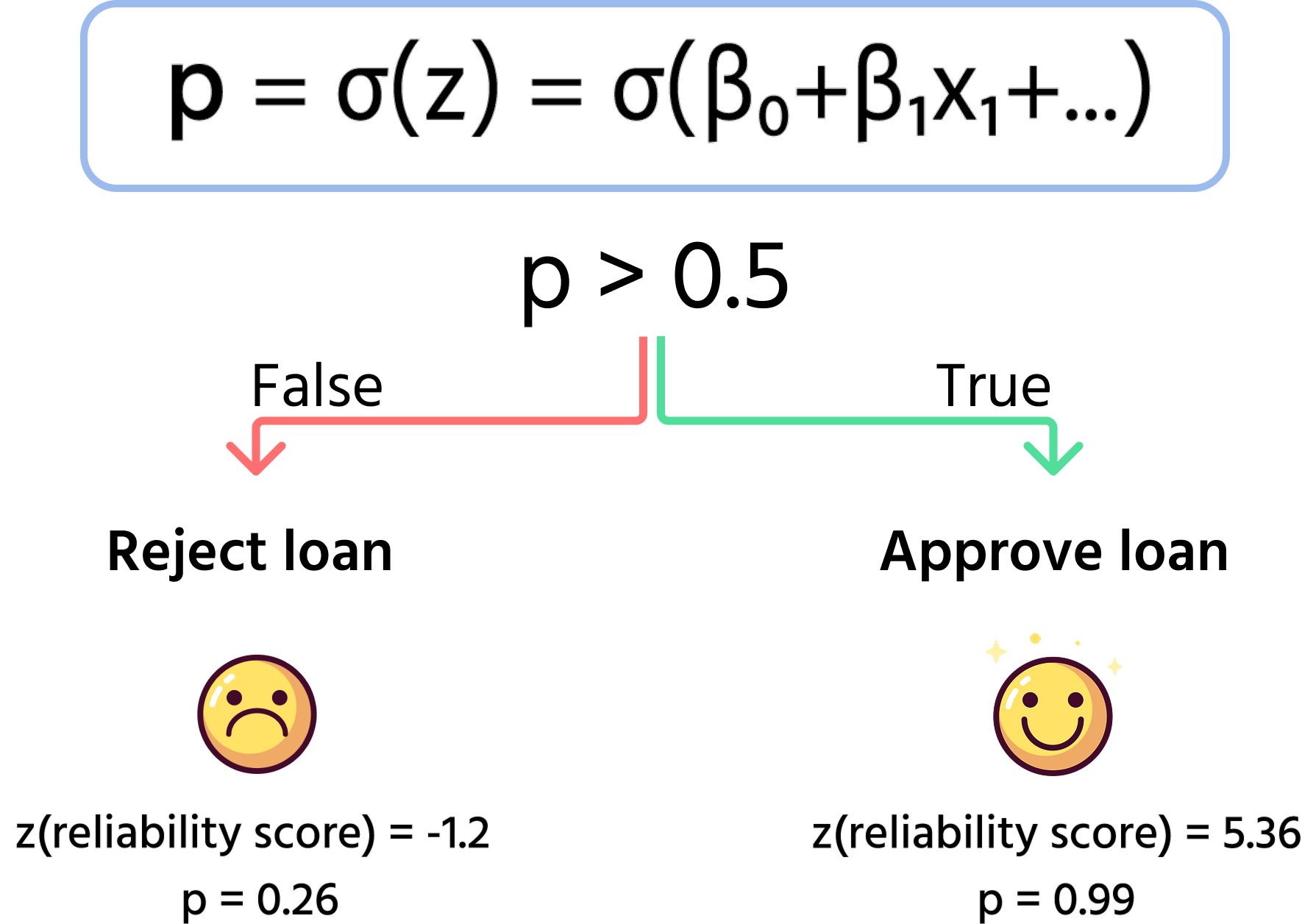Course Content

Classification with Python

##What is Logistic Regression

Although Logistic Regression has the "Regression" in its name, which is a different task, it is the classification algorithm.
Why is it called this way? It is derived from Linear Regression and modified to perform a classification task instead of regression. How is it achieved? Let's look at the example!

Note

This section contains references to a Linear Regression. If you are unfamiliar with Linear Regression, you can check out our Linear Regression with Python course, although you should be fine even without understanding all the references.

Suppose you want to predict whether a person will default on a first loan(no credit history available). In Linear Regression, we built an equation to predict numerical values. We can use the same equation to calculate a "reliability score". It will account for features like income, duration of current employment, debt-to-income ratio, etc. A higher reliability score means a lower chance of default.β's are the parameters the computer will learn during training. The next chapter will cover learning the parameters. For now, let's say we already have the best values of β. The next step would be calculating a reliability score for each instance in the training set and plotting them.

Now we need to convert this score into classes, 0 or 1. The Logistic Regression way to do it is to apply a sigmoid function. It shrinks the predictions to a 0 to 1 range.Now the prediction line we get is a probability of belonging to the class "1".
To convert the prediction to 0 or 1 instead of a probability, we can use the threshold(usually set to 0.5). If the predicted probability is higher than the threshold, we classify the instance as 1, else as 0.We will not dive into the mathematical details of choosing the sigmoid function, nor do you necessarily need to understand its equation. However, looking at the plot, you can see why it suits our task. It confidently predicts the class for a very low or high reliability score. And in the middle, where we have instances from both classes, and we cannot be sure, it predicts a probability around 0.5 that slowly grows as the z gets bigger.

So, that's the idea behind Logistic Regression. Obviously, this approach can be generalized for many different tasks, for example calculating a "cookieness score" to classify sweets as cookie/not a cookie :). But for this whole Logistic Regression thing to work well, we should correctly find the β parameters. That's what you will learn in the next chapter!

#Select all the correct statements.

## Select a few correct answers

Everything was clear?

Section 2. Chapter 1